A Simplified Explanation and Example of SFAS 123R’s Calculated Value Method

We are a small public accounting firm, Accountants CPA Hartford Connecticut, never having any publicly traded clients.  SFAS 123 allowed our nonpublic companies to continue to use the intrinsic value method of APB Opinion No. 25 to value its stock options, only requiring pro forma disclosures for the effect on earnings of using the intrinsic value method instead of the minimum value method as encouraged by the Standard.  Since minimum value could be computed using either a present value computation or an option pricing model, our firm always opted for the former method, avoiding the more mathematically forbidding one.

However, with the implementation of SFAS 123R, the calculated value method is now required for nonpublic companies without an internal market for its stocks or options or for those unable to identify similar public entities for which share or option price information is available.  The implementation of the calculated value method requires the use of an option pricing model and a volatility measure of an appropriate industry sector index.  Like biblical exegesis scholars, our professional staff studied SFAS 123R searching for a comprehensive example of the calculated value method, illustrating in minute detail each step in its calculations of the valuing of a typical stock option of a nonpublic company. Unable to find such, and after spending days attempting to figure out the precise calculations required by eclectically piecing together what we found in the published literature, we decided to publish the results of our efforts in the hope of helping others who might have experienced similar difficulty in implementing the calculated value method and who desired a more comprehensive example illustrating its implementation in its entirety than is found in the Statement.

SFAS 123R states that a lattice model and a closed-form model are valuation techniques meeting its criteria for estimating fair values of employees share options, specifically mentioning the binomial model and the Black-Scholes-Merton formula as examples of each, respectively.  Although the Statement does not specify a preference for a particular valuation technique, as our option pricing model, we chose the Black-Scholes-Merton model to illustrate the calculated value method because not only is it the most widely known option-pricing model, but companies have rarely used the binomial model to measure option values under SFAS No. 123.

The Black-Scholes-Merton formula is not given in SFAS 123R.  The formula that we are employing in this illustration is the following:Here, the assumptions under the option-pricing model are the following:

S = the current price of the underlying share.

E = the exercise price of the option.

t = the expected term of the option.= the expected dividends on the stock for the expected term of the option.

r = the risk-free interest rate for the expected term of the option.

σ = the expected volatility of the price of the stock for the expected term of the option.

Typically the first three assumptions are fairly obvious and not worth further discussion; however, the latter three require further elaboration.

The expected dividends on the stock is expressed as a continuously compounded annual percentage of stock price.  To arrive at a continuously compounded rate, one must take the natural logarithm of 1 plus the rate, k:  i.e.,= ln(1+k)

Similarly, the risk-free rate, r, also has to be expressed as a continuously compounded yield for the Black-Scholes-Merton model.  For a closed-form model as the Black-Scholes-Merton formula, SFAS 123R requires that the risk-free interest rate be “the implied yield currently available on U.S. Treasury zero-coupon issues with a remaining term equal to the expected term….”  One can find the appropriate risk-free rate in The Wall Street Journal under U.S. Treasury Strips.  Again, one must take the natural logarithm of 1 plus the rate, which is the U.S. Treasury zero-coupon yield, y:  i.e., r = ln(1 + y)

Perhaps the most difficult variable to derive for the calculated value method of the pricing-option model involves the expected volatility.  Here, expected volatility is the annualized standard deviation of the differences in the natural logarithms of the comparable industry sector indices for a period of time equal in length to the expected term of the option prior to the grant date.  For example, if the differences in the natural logarithms of the periodic observations of the index is ln(In/In-1), then their standard deviation is represented as follows:The annualized standard deviation is simply the product of the standard deviation as computed above times the square root of the number of periodic observations of the index in a year.  If daily observations are used in the derivation of the standard deviation, simply multiply it by the square root of 260.

The historical values of the Dow Jones industry sector indexes can be obtained from the website, http://www.djindexes.com/.First one would click on “classification” on the top bar to determine the subsector of the company whose options are being valued.  Then one would select under “Benchmark Indexes” “TMI – U.S./World Markets.”After choosing “Index Data, under “historical values” specify the appropriate criteria:  country; sector; market cap range; currency; beginning and ending dates equivalent to the life of the option utilizing the most currently available date; and frequency.After generating the report, “view the table in excel.”With the index data downloaded into an Excel spreadsheet, add an adjacent column computing the closing value ratio for each closing value:  i.e., closing valuei /closing valuei-1.  In the next adjacent column, insert the natural logarithms of the closing value ratios just computed:  i.e., = ln(closing valuei /closing valuei-1).  By inserting the Excel function, =stdev(ln(closing valuei /closing valuei-1), ln(closing valuei+1/closing valuei),….), to compute the standard deviation of all of the natural logarithms of the ratios of the closing values,  and then by multiplying the resultant standard deviation by the square root of 260 (assuming daily observations were used), one calculates the annualized the expected volatility of the price of the stock for the expected term of the option.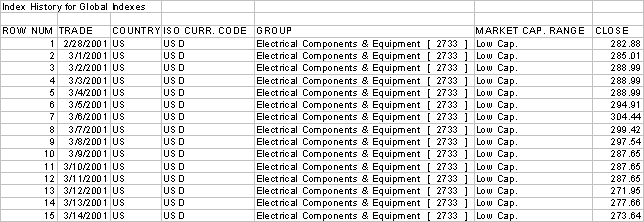Assume a wire and cable company grants 100 options on February 28, 2006, allowing employees to purchase its stock at its current price of \$3,000 over a five-year term.  Further assume that the company does not have an active market for its shares, and that there are no sufficiently comparable companies from which it could derive the value of its options.

Further assume that the company has offered the following dividends over the past ten years:

 Year 1996 1997 1998 1999 2000 2001 2002 2003 2004 2005 Dividend 100 110 125 130 100 100 110 125 130 135 Price 2000 2300 2700 3000 2200 2300 2600 2700 2800 3000 % 5.00% 4.78% 4.63% 4.33% 4.55% 4.35% 4.23% 4.63% 4.64% 4.50%

The average dividend percentage is 4.56%; and its continuously compounded rate is computed as follows: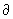= ln(1.0456), or 4.463%.

Looking in the Wall Street Journal on February 28, 2006 for the U.S. Treasury zero-coupon yield for an instrument maturing on February 28, 2011, one would find a yield of 4.608%.  The risk-free rate, a continuously compounded rate, is computed as follows:

r = ln(1.04608), or 4.505%.

To compute the expected volatility of the price of the company’s stock, on the website http://www.djindexes.com/, after selecting TMI – U.S./World Markets on the main webpage and Index Data on the following webpage as illustrated above, highlight USA as the country, Electrical Components & Equipment 2733 as the sector, Small Cap as the Market Cap Range, US Dollar as the Currency Selection, Begin Date as February 28, 2001, End Date as February 28, 2006, and Frequency as Daily.

Downloading the table in Excel, adding columns for the ratios of closing values and for their natural logarithms, and computing the annualized standard deviation are illustrated below:~                           ~                           ~                           ~

~                           ~                           ~                           ~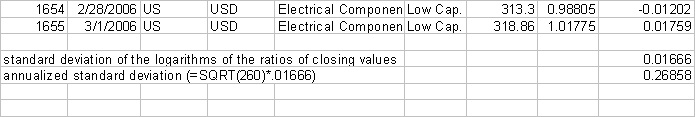From the above calculation, one has derived the expected volatility, σ, of the price of the stock for the expected five-year term of the option to be .26858.

Now one is ready to insert the values of the variables into the Black-Scholes-Merton formula.  First, solve for:Using Excel, we solve for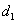:

 =(LN(3000/3000)+(0.04505-0.04463+(0.26858^2)/2)*5)/0.26858*SQRT(5)

or,= .30378.

Since, solving foris a matter of simple substitution:- 0.26858*SQRT(5)

or,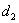= -.29678.

Now plugging these values ofandinto Black-Scholes-Merton formula,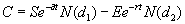, utilizing the following Excel functions for exponential and normal distributions, we derive the value of the options:

 = 3000*EXP(-.04463*5)*NORMSDIST(.30378)-3000*EXP(-.04505*5)*NORMSDIST(-.29678)

= \$568.41,

(where the standard normal distribution of .30378 = .61935, and where the standard normal distribution of -.29678 = .38332).

Consequently, the value of the 100 options issued on February 28, 2006 is \$56,841.

Excel greatly simplifies the above calculations by providing readily computed values of the standard deviation and the standard normal distributions, which otherwise would require innumerable entries into a statistical calculator and the use of standard normal tables.

Saving the Excel representation of the Black-Scholes-Merton formula and inserting the six requisite assumptions under the option-pricing model (i.e., current price, exercise price, expected term, expected dividend rate, risk-free interest rate, and the expected volatility of the price of the stock) into the above illustrated Excel formula should help demystify the computation of option prices utilizing the calculated value method for any staff or, for that matter, any managerial member of a public accounting firm, as it did at ours.  If you have any comments, suggestions, or questions, please visit our website: http://www.cpa-connecticut.com.

Article written by William Brighenti, CPA, CVA, Certified QuickBooks ProAdvisor, Sage Master Builder Consultant, Director of Accountants CPA Hartford Connecticut.

Accountants CPA Hartford    Construction Accountants CPA Hartford    About Accountants CPA Hartford    Accountants CPA Hartford Accounting Services    Contact Accountants CPA Hartford    Accountants CPA Hartford Accounting & Tax Tips    Articles of Accountants CPA Hartford    IPIC Method Illustrated    Accountants CPA New Britain    Not-for-Profit Accountants CPA Hartford CT